Picture by "Hello, I'm Nik", Unsplash.

Charts and plots can often look a bit stale and professional, which might not be appropriate in every setting. If you want to dumb-down your charts and make them look a bit friendlier and less intimidating for your target audience, you might want to consider CuteCharts.

This quirky Python package works with Pandas and allows you to produce charts and plots with a unique hand-drawn look, and includes bar plots, line plots, pie charts, radar charts, and scatter plots. Here’s how it works.

To get started, open a Jupyter notebook and load up the packages below. We’ll be using Pandas to create some data to visualise, and CuteCharts to create the plots and graphs. You can install CuteCharts by typing `pip3 install cutecharts` into your terminal.

``````import pandas as pd
from cutecharts.charts import Bar
from cutecharts.charts import Pie
from cutecharts.charts import Line
from cutecharts.charts import Scatter
from cutecharts.components import Page
``````

#### Create some dataframes

Next, we’ll create a couple of dataframes containing some simple data to plot…

``````data = {'Lord': ['Lord of the Rings','Lord of the Flies','Lord of the Dance'],
df_lords = pd.DataFrame(data, columns = ['Lord', 'Votes'])
``````
0 Lord of the Rings 2121
1 Lord of the Flies 7885
2 Lord of the Dance 10195
``````data = {'Day': ['Mon', 'Tue', 'Wed', 'Thu', 'Fri', 'Sat', 'Sun'],
'This week': [12, 10, 9, 9, 10, 3, 3],
'Last week': [15, 12, 8, 9, 11, 4, 3]
}
df_coffee = pd.DataFrame(data, columns = ['Day', 'This week', 'Last week'])
``````
Day This week Last week
0 Mon 12 15
1 Tue 10 12
2 Wed 9 8
3 Thu 9 9
4 Fri 10 11

#### Create a bar chart

Creating plots in CuteCharts is slightly more long-winded than doing it in Seaborn, but still quicker than bog-standard Matplotlib. First, we’ll instantiate a new `chart` set the to `Bar()` type and we’ll assign a title that we’ll set to display in uppercase letters.

Then we’ll define the chart options using the `set_options()` function. This requires the column of labels from our `df_lords` dataframe, a name for the `x_label`, and a name for the `y_label`.

Finally, we add a series of data containing the votes, and use the `render_notebook()` function to display the chart. CuteCharts are JavaScript based, so you can hover over them to see tooltips containing the raw metrics.

``````chart = Bar(str.upper('Which is the best Lord?'))
chart.set_options(
labels=list(df_lords['Lord']),
x_label='Lord',
)
chart.render_notebook()
``````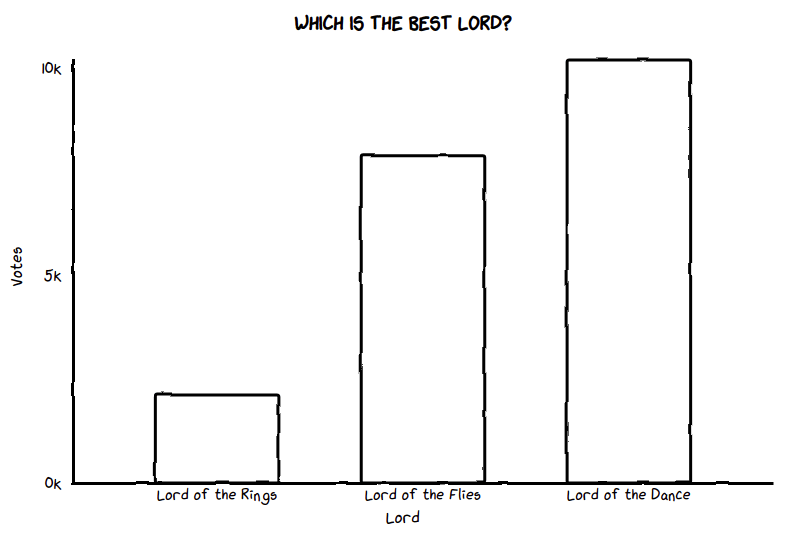#### Create a pie chart

We can use the same dataframe of Lords to create a pie chart. This only requires the `labels` containing each Lord’s name, plus an `inner_radius` value, which can be set to a value over zero to turn the pie into a donut.

``````chart = Pie(str.upper('Which is the best Lord?'))
chart.set_options(
labels=list(df_lords['Lord']),
)
chart.render_notebook()
``````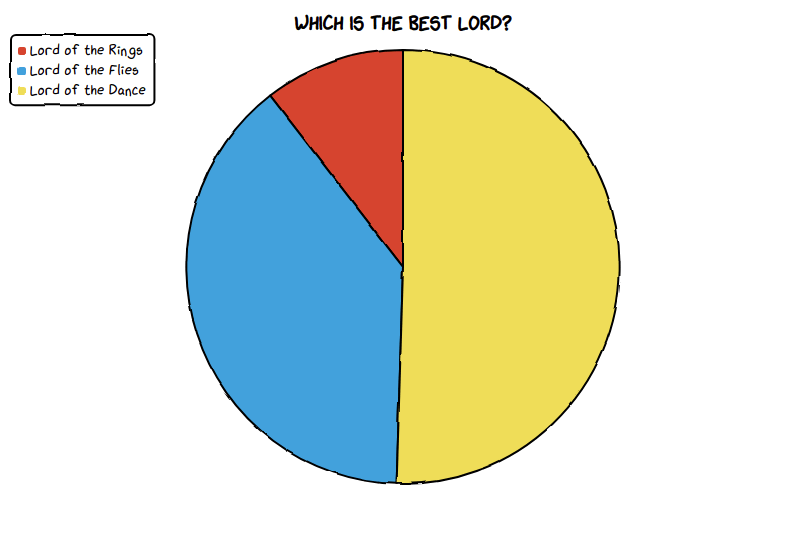#### Create a line chart

For the line chart we’ll use the coffee consumption dataframe. The concept is similar to the bar chart, except that we add multiple series’ of data containing each dataframe series or column that we want to plot.

``````chart = Line('Cups of coffee consumed per day')
chart.set_options(
labels=list(df_coffee['Day']),
x_label='Day',
y_label='Cups of coffee'
)
chart.render_notebook()
``````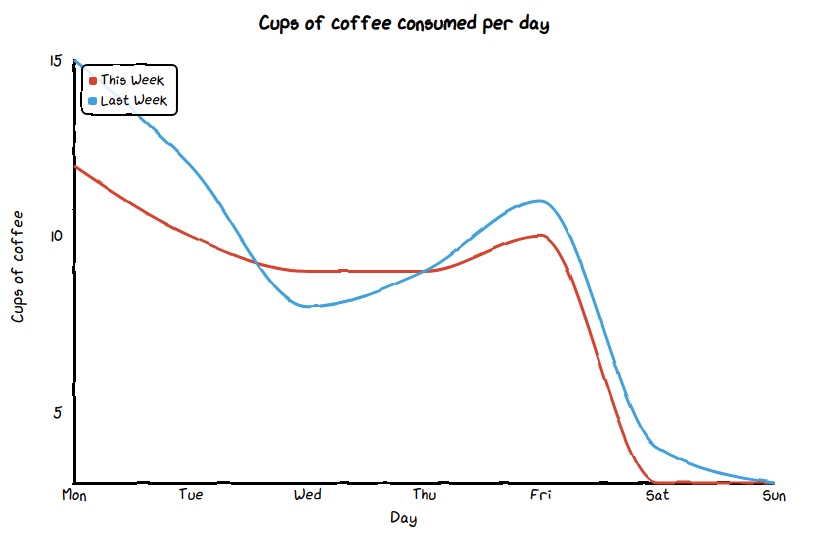The radar plot can also be produced using the coffee consumption dataset. With this one, we’re manually defining the legend position as the upper right of the page.

``````chart = Radar('Cups of coffee consumed per day')
chart.set_options(
labels=list(df_coffee['Day']),
is_show_legend=True,
legend_pos='upRight'
)
chart.render_notebook()
``````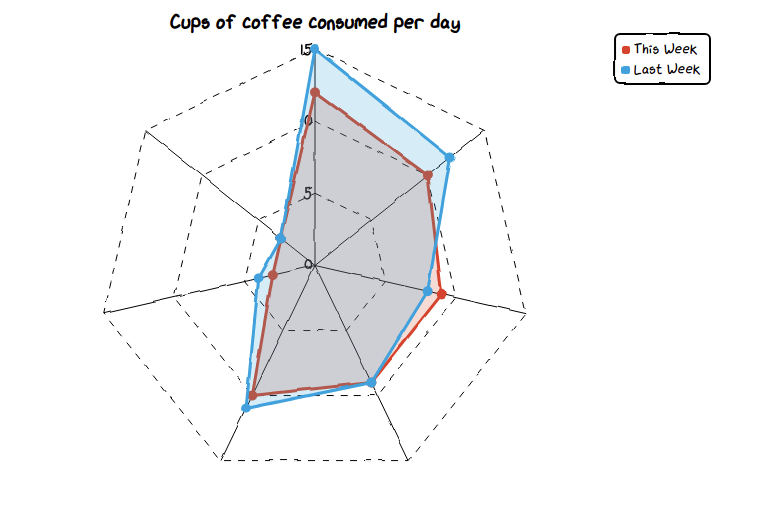#### Create a scatter plot

Finally, we can create a simple scatter graph or scatter plot using `Scatter()`. Here, we need to provide `set_options()` with the `x_label`, `y_label`, and an optional custom colour for the dots. The `add_series()` function then takes a `zip()` of the two metrics, so we can plot any relationship between the two.

``````suicide_rate = [14.2, 16.4, 11.9, 15.2, 18.5, 22.1, 19.4, 25.1, 23.4, 18.1, 22.6, 17.2]
ie_usage     = [215, 325, 185, 332, 406, 522, 412, 614, 544, 421, 445, 408]

chart = Scatter('Internet Explorer usage versus Suicide rate')
chart.set_options(
x_label='Suicide rate',
y_label='Internet Explorer usage',
colors=['#009989'],
)
chart.add_series('Suicide rate', [(z, z) for z in zip(suicide_rate, ie_usage)])
chart.render_notebook()
``````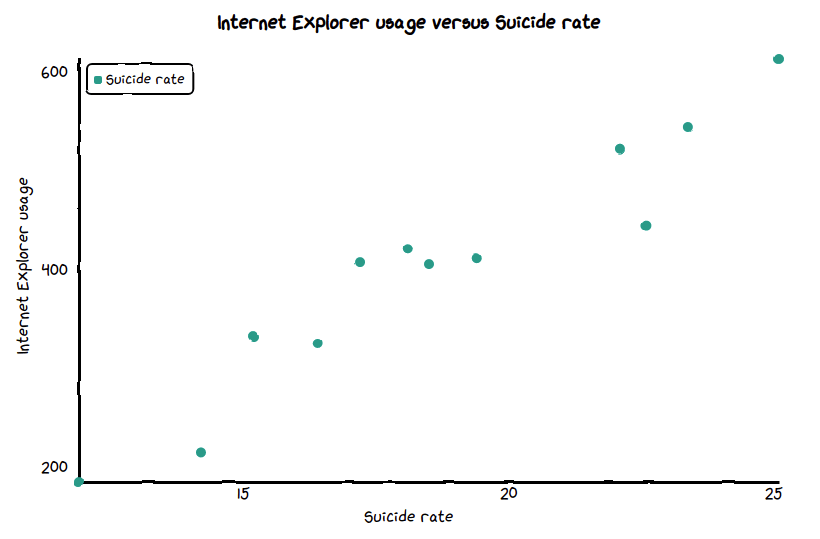Matt Clarke, Monday, March 08, 2021

Matt Clarke Matt is an Ecommerce and Marketing Director who uses data science to help in his work. Matt has a Master's degree in Internet Retailing (plus two other Master's degrees in different fields) and specialises in the technical side of ecommerce and marketing.